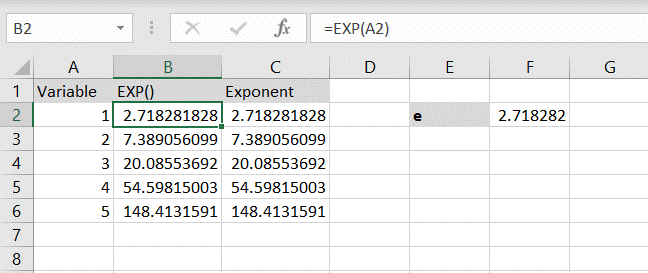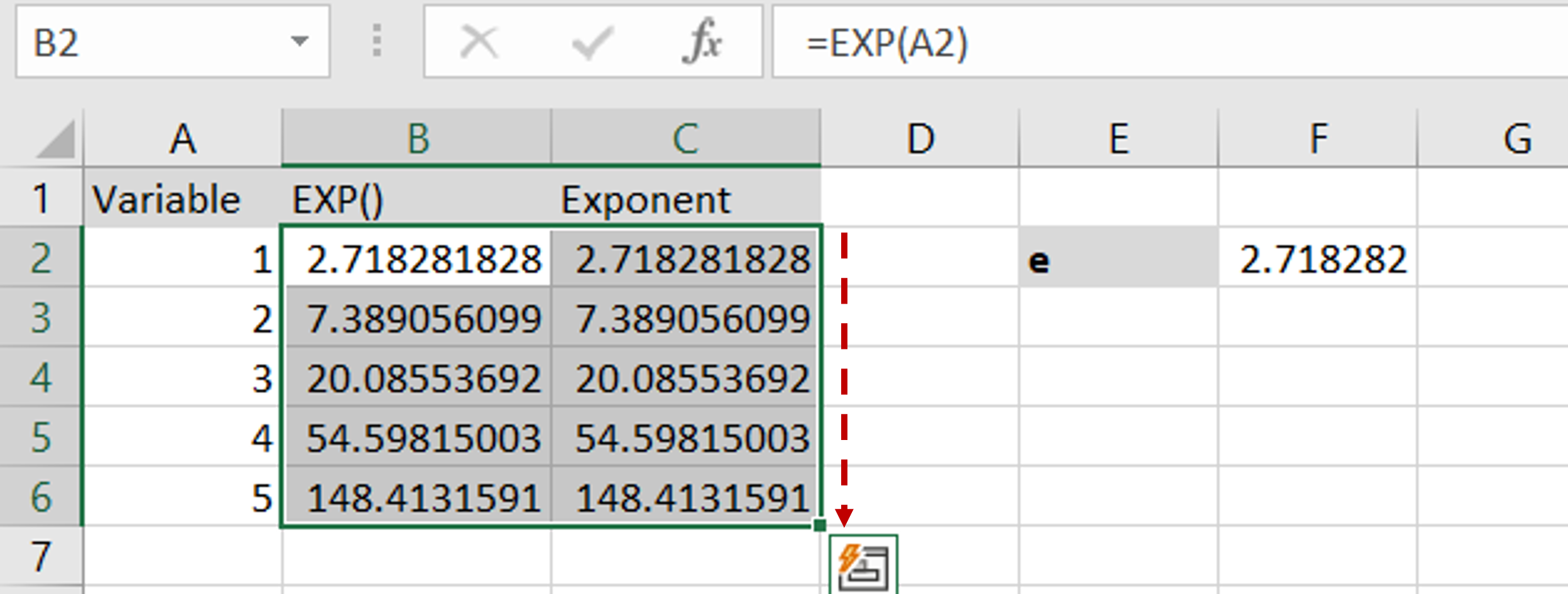# How to do e^ in Excel

You can watch a video tutorial here.The symbol ‘e’ stands for Euler’s number and is the base for natural logarithms. It is approximately 2.71828182845904. In Excel, to use e you can use the EXP() function. This will raise e to the power of the number. The syntax of EXP() is as follows:

EXP(number)
– number: the number that e is to be raised to

To demonstrate the use of e, we will compare it with using the exponent operator (^) in Excel which will also raise e to the power of a number.

### Step 1 – Create the EXP() formula– In the EXP() column, type the formula using cell references:
=EXP(Variable)

### Step 2 – Create the exponent formula– In the exponent column, type the formula using cell references:
=\$e\$^Variable
– Dollar signs are added to the cell reference for e, to make it constant, by selecting it and pressing F4

### Step 3 – Copy the formula and check the result– Using the fill handle from the first cell, drag the formula to the remaining cells
OR
a) Select the cell with the formula and press Ctrl+C or choose Copy from the context menu (right-click)
b) Select the rest of the cells in the column and press Ctrl+V or choose Paste from the context menu (right-click)
– Both methods return the same result